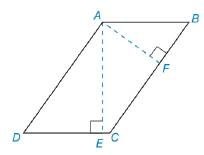Chapter 8.1, Problem 50EElementary Geometry For College St...

7th Edition
Alexander + 2 others
ISBN: 9781337614085

Solutions

Chapter
SectionElementary Geometry For College St...

7th Edition
Alexander + 2 others
ISBN: 9781337614085
Textbook Problem

In Exercises 47 to 50, use the fact that the area of the polygon is unique.In ▱ A B C D ,  AB = 7 and B C = 12. The length of altitude A F ¯ (to side B C ¯ ) is 5. Find the length of altitude A E ¯ from A to D C ¯ .To determine

To find:

The length of altitude

Explanation

Converting theoretical statement into mathematical statement, identifying the geometric figure and the calculation is done by suitable geometric formulae.

Calculation:

Given,

Here, BC=b=12 and AF=h=5

Area of the parallelogram A=bh unit2.

A=(12)(5)

A=60 unit2.

Find AE:

For A to DC¯

Consider DC¯=b

Still sussing out bartleby?

Check out a sample textbook solution.

See a sample solution

The Solution to Your Study Problems

Bartleby provides explanations to thousands of textbook problems written by our experts, many with advanced degrees!

Get Started

Expand each expression in Exercises 122. (4y2)y

Finite Mathematics and Applied Calculus (MindTap Course List)

True or False: If , then converge absolutely.

Study Guide for Stewart's Multivariable Calculus, 8th

The quadratic approximation for at a = 1 is:

Study Guide for Stewart's Single Variable Calculus: Early Transcendentals, 8th